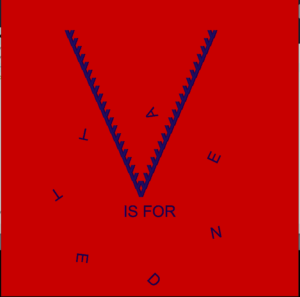# Generative Text

For this week’s project, I decided to base my work on a popular movie, an old one though: V for Vendetta. My work, which uses the manipulation of text, is divided into two parts.

The first part is a big V, but only if you look closely can you see the detail. In a nested for loop, I made the V so that every line would have three “v”s, with each character being less transparent than the one before. This, however, doesn’t show much since the characters are two close together but if you look closely you might spot a slight color difference.

For the next part, I made a simple rotation scheme where I would have it so that the big V would stay there but the characters around it would orbit in a circle so that it would altogether spell out “Vendetta”.

```PFont myFont;
char v = 'v';
int z = 15;
int x = 100;
int y = 100;
float a = 400;
float b = 500;
float r = 30;
String s = "ENDETTA";
void setup(){
size(750,750);
background(200,0,0);
myFont = createFont("Helvetica",40);
textFont(myFont,40);
textAlign(CENTER);
}

void draw(){

int x = 170;
int y = 100;
for(int i=0;i<20;i++){

for(int j=0;j<3;j++){
//println(z);
color a = color(19,24,98,z);
fill(a);
text(v,x,y);
x += 3;
z -= 5;

}
z = 15;

y += 20;

}
for(int i=0;i<21;i++){

for(int j=0;j<3;j++){
//println(z);
color a = color(19,24,98,z);
fill(a);
text(v,x,y);
x += 3;
z -= 5;

}
z = 15;

y -= 20;

}

text("IS FOR",375,550);
a = 400;
b = 500;
float mb = 0;
//pushMatrix();
//translate(400,500);
translate(350,500);
for(int i=0;i<s.length();i++){
char d = s.charAt(i);
float h = textWidth(d);

mb += h/2;
float theta = PI + mb/r;

pushMatrix();
translate(r*cos(theta),r*sin(theta));
rotate(theta+PI/2);
text(d,170,170);
popMatrix();
mb += h/2;

}
//popMatrix();

}```

Paintings speak better than words. Here is my final creation: